# Banked Curve Formulas & Circular Motion

Cynthia Helzner, Chelsea Schuyler
• Author
Cynthia Helzner

Cynthia Helzner has tutored middle school through college-level math and science for over 20 years. She has a B.S. in microbiology from The Schreyer Honors College at Penn State and a J.D. from the Dickinson School of Law. She also taught math and test prep classes and volunteered as a MathCounts assistant coach.

• Instructor
Chelsea Schuyler

Chelsea has a bachelor's degree in biology and works in online content writing.

Learn what a banked curve is. Study formulas for banking circular motion with and without friction, and discover solved examples of banked curve problems. Updated: 03/14/2022

Show

## Motion on a Circular Track

Uniform circular motion is movement in which an object is moving at a constant speed in a circular path. Motion on a circular track without speeding up or slowing down is thus a type of uniform circular motion.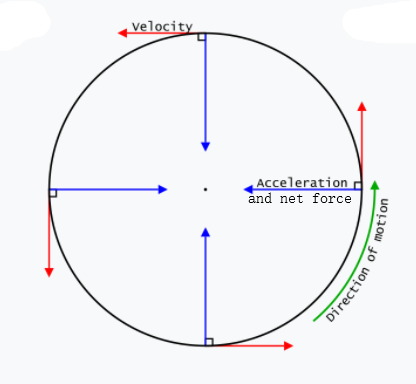Although the speed in uniform circular motion is constant, the velocity is not constant because velocity is a vector (i.e. it has both magnitude and direction) and the direction of motion is constantly changing as the object goes around the circle. The force causing that change in direction is directed centripetally (toward the center of the circle). Accordingly, that force is called the centripetal force.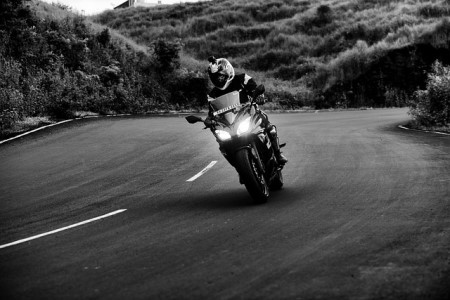## On-Ramp

When entering a curved section of any road, you might notice the road isn't flat, but banked. A banked surface is one that slopes upward towards the outer edge of a curve. Banked surfaces exist at on-ramps to highways because cars have to start traveling faster to enter the highway. The angle of banked surfaces of race car tracks range from 12° to 36°.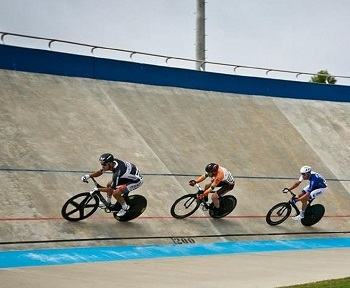Let's go through the derivation of equations involving circular motion on banked surfaces.

## What is a Banked Curve?

Uniform circular motion driving problems can take place on unbanked (flat) or banked curves. So, what is a banked curve? A banked curve is a turn in which the driving surface is not horizontal. Banked curves in roads and racetracks are tilted inward (i.e. toward the center of the circle) in order to help vehicles get around the turn. Figure 1 shows an unbanked curve, while Figure 2 shows a banked track.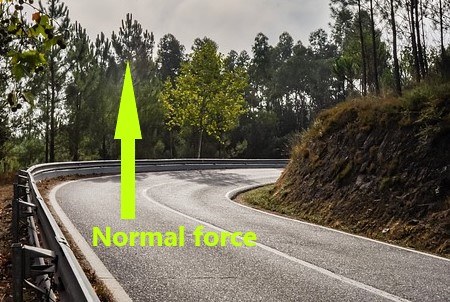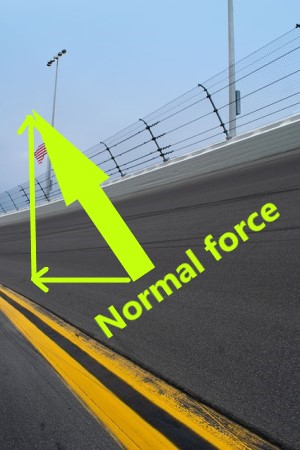In an unbanked curve, the centripetal force is only provided by the force of friction between the vehicle's tires and the road because the normal force (the reaction "push" force that is perpendicular to the surface) is vertical. In contrast, a banked curve provides a tilted normal force, so the normal force has a horizontal component that contributes to the centripetal force. Particularly when roads are icy (which decreases the force of friction between the vehicle's tires and the road), this horizontal component is crucial to having enough centripetal force to change the direction of the vehicle quickly enough for it to safely round the turn without slipping.

## Formulas for Banking Circular Motion

The formulas (other than object height) for banking circular motion depend on whether friction is being included or ignored. In the formulas below, the Greek letter {eq}\mu {/eq} means the coefficient of friction, which is a measure of how strong the force of friction is between two particular surfaces. {eq}\mu {/eq} is unitless. When friction is being ignored, {eq}\mu = 0 {/eq} and the equations simplify accordingly.

### With Friction

When the velocity of the object is more than the optimal velocity, the object will be prone to sliding up the incline; so the force of friction (which always opposes the direction of motion) will be down the incline. When the velocity of the object is less than the optimal velocity, the object will be prone to sliding down the incline; so the force of friction will be up the incline. Either way, friction will affect the net centripetal force and normal force experienced by the object. The force of gravity is always downward, whether the normal force is vertical or not.

In the equations below, the Greek letter {eq}\theta {/eq} means the angle between the banked surface and the horizontal g is the gravitational constant ({eq}9.8 \frac{m}{s^2} {/eq}), v is velocity in meters per second, h is the vertical height of the object in motion, s is the optimum speed in meters per second, d is how far diagonally up the track the object is, and r is the radius of the circle in meters.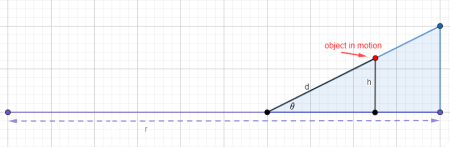• banking angle formula: {eq}tan\theta = \frac{\frac{v^2}{rg} - \mu}{1 + \frac{\mu v^2}{rg}} {/eq} or {eq}\theta = tan^{-1}\left(\frac{\frac{v^2}{rg} - \mu}{1 + \frac{\mu v^2}{rg}}\right) {/eq}
• object height formula: {eq}h = dsin\theta {/eq}
• optimum speed formula: {eq}s = \sqrt{\frac{rg(tan\theta + \mu)}{1 - \mu tan\theta}} {/eq}

### Without Friction

When friction is being ignored, treat {eq}\mu {/eq} as if it were 0. Doing so simplifies the equations significantly and makes the horizontal component of the normal force be the only source of the centripetal force. As before, {eq}\theta {/eq} is the banking angle, g is the gravitational constant ({eq}9.8 \frac{m}{s^2} {/eq}), v is velocity in meters per second, h is the vertical height of the object in motion, s is the optimum speed in meters per second, d is how far diagonally up the track the object is, and r is the radius of the circle in meters.

• banking angle formula: {eq}tan\theta = \frac{v^2}{rg} {/eq} or {eq}\theta = tan^{-1}\left(\frac{v^2}{rg}\right) {/eq}
• object height formula: {eq}h = dsin\theta {/eq}
• optimum speed formula: {eq}s = \sqrt{rgtan\theta} {/eq}

### Banked Curves and Friction

Banked curve problems will either account for or ignore friction, so be sure to choose the correct version of the above formulas. For problems with friction, any necessary value of {eq}\mu {/eq} should be provided in the problem or in a reference table because that value changes based on the surfaces involved.

Remember that when the velocity of the object is more than the optimal velocity, the force of friction is down the incline. When the velocity of the object is less than the optimal velocity, the force of friction will be up the incline.

## Banked Curve Formula Examples

Here are some examples of banked curve problems that use the equations described earlier in this article. These examples cover both with-friction and without-friction contexts.

Example 1 (banking angle without friction)

A highway engineer needs to figure out the banking angle for a curve with radius 210 m, at which cars of mass 2000 kg will be traveling at {eq}28 \frac{m}{s} {/eq} in icy conditions (i.e. friction will be negligible). What is the banking angle?

First, choose the relevant equation. The banking angle without friction is {eq}\theta = tan^{-1}\left(\frac{v^2}{rg}\right) {/eq}. Note that this equation does not contain m so, even though mass was given in the problem, it will not be used here.

Next, list the values needed for the equation: v = {eq}28 \frac{m}{s} {/eq}, r = 210 m, and g is the gravitational constant ({eq}9.8 \frac{m}{s^2} {/eq}).

Finally, insert those values into the equation: {eq}\theta = tan^{-1}\left(\frac{28^2}{210\cdot 9.8}\right) = 21^{\circ} {/eq}.

Example 2 (banking angle with friction)

What should the banking angle be for a racetrack curve with radius 0.20 km at which cars will be traveling at {eq}61 \frac{m}{s} {/eq} with a coefficient of friction of 0.70?

First, notice that r is not given in meters, so convert it: 0.20 km = 200 m.

Then, choose the relevant equation. The banking angle with friction is {eq}\theta = tan^{-1}\left(\frac{\frac{v^2}{rg} - \mu}{1 + \frac{\mu v^2}{rg}}\right) {/eq}.

Next, list the values needed for the equation: v = {eq}61 \frac{m}{s} {/eq}, r = 200 m, {eq}\mu {/eq} = 0.70, and g = {eq}9.8 \frac{m}{s^2} {/eq}.

Finally, insert those values into the equation: {eq}\theta = tan^{-1}\left(\frac{\frac{61^2}{200\cdot9.8} - 0.70}{1 + \frac{0.70\cdot61^2}{200\cdot9.8}}\right) = 39^{\circ} {/eq}.

Example 3 (object height without friction)

How high above the bottom of the track is a skateboarder who is going around a 45° banked track when the skateboarder is 12 m up the slope of the track? Ignore friction.

First, choose the relevant equation. The object height equation with or without friction is {eq}h = dsin\theta {/eq}.

Next, list the values needed for the equation: d = 12 m and {eq}\theta {/eq} = 45°.

Finally, insert those values into the equation: {eq}h = 12sin(45^{\circ}) {/eq} = 8.5 m.

Example 4 (object height with friction)

How high above the bottom edge of the road is a truck that is going around a 15° banked curve when the truck is 2 m up the slope of the road with a coefficient of friction of 0.75?

First, choose the relevant equation. The object height equation with or without friction is {eq}h= dsin\theta {/eq}. This equation does not contain {eq}\mu {/eq} so, even though coefficient of friction is given, it is not needed for this problem.

Next, list the values needed for the equation: d = 2 m and {eq}\theta {/eq} = 15°.

Finally, insert those values into the equation: {eq}h = 2sin(15^{\circ}) {/eq} = 0.5 m.

Example 5 (optimal speed without friction)

## Derivation of Bank Angle

Let's start with the derivation of the angle of a banked surface. This has to be based on the radius of the circular path, and the speed of the object on that surface without friction. Diagram 1 shows the angle of bank, the radius of the curve, and how high the object is from the flat surface.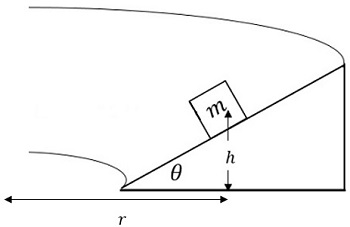We have to analyze the forces that are at play in this scenario. At the optimal speed, angle, and radius of curvature, no friction is required. Diagram 2 shows the forces on the mass.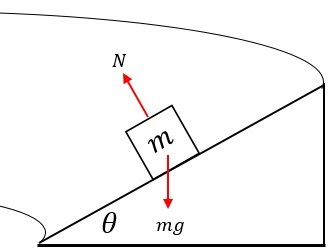Newton's second law is ΣF = ma, and the Σ tells us we need to add all of the forces acting on an object to determine its acceleration. The acceleration in our scenario is centripetal, which means it is acting towards the center of the circle. The centripetal acceleration equation is: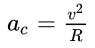where

• ac is the centripetal acceleration in meters-per-second-squared (m/s2)
• v is velocity in meters-per-second (m/s)
• r is the radius of the circle in meters (m)

Now we can plug this equation into Newton's second law giving us Equation 1.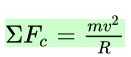Forces are vectors, which means they must be resolved into component directions. We have to set an x-y axis system, and resolve the forces. Diagram 3 shows the resolution of the normal force.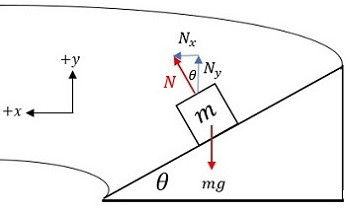The normal force's x and y-components are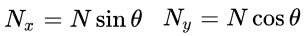The weight is already in the pure y-direction.

The x-component of the normal force is what is causing the centripetal acceleration. Let's plug it into Equation 1, giving us Equation 2.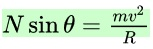We don't want the normal force in our equation, so we use the expression derived from the y-direction forces to substitute for it. Adding the y-direction forces gives us: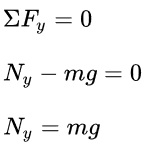Notice the normal force is still there. The substitute for Ny is the weight. We will call this Equation 3.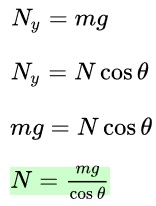The last step is to plug Equation 3 into Equation 2, which will give us an expression for the tangent of the angle of the banked surface for an object traveling at velocity v with radius r. We'll call this Equation 4.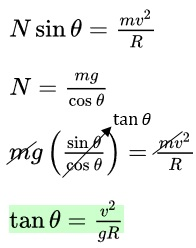## Height of Object in Banked Curve

Since we have a right triangle, we can use the Pythagorean theorem along with Equation 4 to determine the height of the object from the level surface. Diagram 4 shows the height of the mass on the banked surface.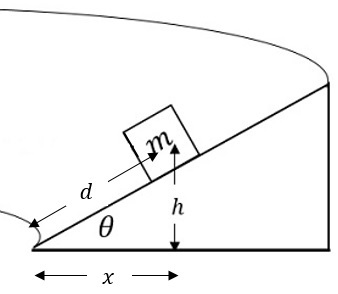First, lets use the Pythagorean theorem to get an expression for x, which we'll call Step 1.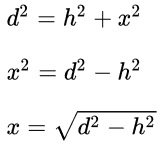Let's update Diagram 4 to show the new expression for x.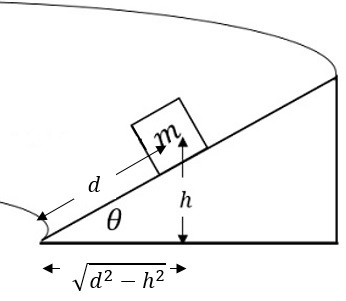Now we can equate tan θ from the right triangle with Equation 4 giving us Step 2.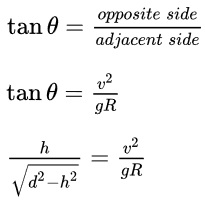When looking at a banked road, you can see the height is very small compared to the distance from the mass to the bottom of the surface (hypotenuse), therefore we can ignore the h2 term. This gives us Equation 5.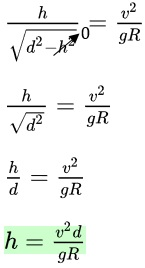## Optimum Speed

In Equation 4, the bank angle, velocity and radius are the only variables. We can determine the optimum speed of a mass moving along a banked surface by solving Equation 4 for velocity.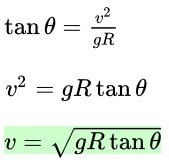To unlock this lesson you must be a Study.com Member.

## On-Ramp

When entering a curved section of any road, you might notice the road isn't flat, but banked. A banked surface is one that slopes upward towards the outer edge of a curve. Banked surfaces exist at on-ramps to highways because cars have to start traveling faster to enter the highway. The angle of banked surfaces of race car tracks range from 12° to 36°.Let's go through the derivation of equations involving circular motion on banked surfaces.

## Derivation of Bank Angle

Let's start with the derivation of the angle of a banked surface. This has to be based on the radius of the circular path, and the speed of the object on that surface without friction. Diagram 1 shows the angle of bank, the radius of the curve, and how high the object is from the flat surface.We have to analyze the forces that are at play in this scenario. At the optimal speed, angle, and radius of curvature, no friction is required. Diagram 2 shows the forces on the mass.Newton's second law is ΣF = ma, and the Σ tells us we need to add all of the forces acting on an object to determine its acceleration. The acceleration in our scenario is centripetal, which means it is acting towards the center of the circle. The centripetal acceleration equation is:where

• ac is the centripetal acceleration in meters-per-second-squared (m/s2)
• v is velocity in meters-per-second (m/s)
• r is the radius of the circle in meters (m)

Now we can plug this equation into Newton's second law giving us Equation 1.Forces are vectors, which means they must be resolved into component directions. We have to set an x-y axis system, and resolve the forces. Diagram 3 shows the resolution of the normal force.The normal force's x and y-components areThe weight is already in the pure y-direction.

The x-component of the normal force is what is causing the centripetal acceleration. Let's plug it into Equation 1, giving us Equation 2.We don't want the normal force in our equation, so we use the expression derived from the y-direction forces to substitute for it. Adding the y-direction forces gives us:Notice the normal force is still there. The substitute for Ny is the weight. We will call this Equation 3.The last step is to plug Equation 3 into Equation 2, which will give us an expression for the tangent of the angle of the banked surface for an object traveling at velocity v with radius r. We'll call this Equation 4.## Height of Object in Banked Curve

Since we have a right triangle, we can use the Pythagorean theorem along with Equation 4 to determine the height of the object from the level surface. Diagram 4 shows the height of the mass on the banked surface.First, lets use the Pythagorean theorem to get an expression for x, which we'll call Step 1.Let's update Diagram 4 to show the new expression for x.Now we can equate tan θ from the right triangle with Equation 4 giving us Step 2.When looking at a banked road, you can see the height is very small compared to the distance from the mass to the bottom of the surface (hypotenuse), therefore we can ignore the h2 term. This gives us Equation 5.## Optimum Speed

In Equation 4, the bank angle, velocity and radius are the only variables. We can determine the optimum speed of a mass moving along a banked surface by solving Equation 4 for velocity.To unlock this lesson you must be a Study.com Member.

#### At what angle should the curve be banked?

The equation for the banking angle with friction is theta = arctan{((v^2/(rg) - u)/(1 + uv^2/(rg))}. The equation for the banking angle without friction is theta = arctan{v^2/(rg)}. In the equations, theta is the banking angle, u is the coefficient of friction, g is the gravitational constant (9.8 m/s^2), v is velocity in meters per second, and r is the radius of the circular path in meters.

#### What is the difference between banked and unbanked curves?

In a banked (i.e. tilted) curve, both friction and the horizontal component of the normal force contribute to the centripetal force that keeps the object in its circular path without slipping. In an unbanked (i.e. flat) curve, only friction provides the centripetal force; so it is more difficult to get around that type of curve without slipping, especially in icy conditions.

#### How do banked curves work?

In a banked curve, the normal force is not vertical, so both friction and the horizontal component of the normal force contribute to the centripetal force that keeps the object in its circular path without slipping. If the curve were not banked, the normal force would be vertical; so it would not have a horizontal component.

#### What are banked curves used for?

Banked curves make it safer for vehicles to round a turn, especially at high speeds. In a banked curve (unlike in an unbanked curve), there is a horizontal component of the normal force, which contributes to the centripetal force that keeps the object in its circular path without slipping.

### Register to view this lesson

Are you a student or a teacher?